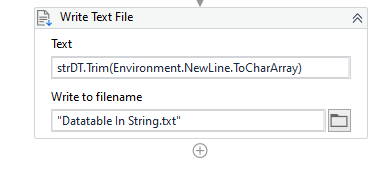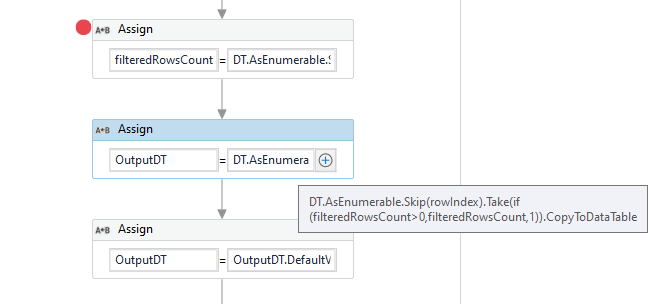# How to read excel value and convert into two dimesional array

Hi all

I need to get CPT codes (f2,f3,f4,f5,f6,f7) and Amount(K2,k3,k4.k5.k6.k7)belong to per claim number(B2) and will save into a string variable with Table format for CPT codes and amount . It may vary per claim numbers

Can anyone please let me know how to do it?

Duplicate post:

Hi @divya.17290 ,

Could you also provide us with the Expected Output format so that we could create the solution logic based on it.

Hi
The following is my Excel sheet

I need to read the values CPT and Amount from the excel sheet by claim number then store into a string variable.
My Expected output is
String Reason :
CPT Amount
1
2 125
3
4 67

Hi @divya.17290 ,

There is a Similar post created by you where we did have the solution received, we could use the same start logic here as well, but here instead of the Array of Strings it is the Table Format that you would require. The post mentioned :

The same logic can be used but the conversion should be to a Datatable.
For that we could change the Linq Expression to the below :

``````filteredRowsCount = DT.AsEnumerable.Skip(rowIndex+1).TakeWhile(Function(x)String.IsNullOrWhiteSpace(x("Payer Claim Number").ToString)).Count
``````

Here, `filteredRowsCount` is a variable to type Integer. We fetch the Total rows from the next position of the row Index of the value found to the next non-empty value. This count is used to retrieve the Filtered/Required rows later.

We could take the Required number of rows since we now have the rowIndex and the number of rows the value found is mapped in the below way :

``````OutputDT = DT.AsEnumerable.Skip(rowIndex).Take(filteredRowsCount).CopyToDataTable
``````

Here, `OutputDT` is a new Datatable.

We now have the filtered rows, but we would need to filter the columns as well, For that we could do the following :

``````OutputDT = OutputDT.DefaultView.ToTable(false,"CPT","Amount")
``````

As we need only `CPT` and `Amount` columns.

Next, in order for the Formatting to be properly made look as a Table, we would need to fetch the count/Length of the Largest word in the Whole Filtered Table (including column Names). For that we could do the following operations :

``````columnNamesLongestValueLength = OutputDT.Columns.Cast(Of System.Data.DataColumn).Max(Function(x)x.columnName.Length)

rowValuesLongestLength = OutputDT.AsEnumerable.Max(Function(x)x.ItemArray.Max(function(y)y.ToString.Length))

LongestLengthValue = if(columnNamesLongestValueLength<rowValuesLongestLength,rowValuesLongestLength,columnNamesLongestValueLength)
``````

Here, the variables `columnNamesLongestValueLength`, `rowValuesLongestLength` and `LongestLengthValue` are of integer type.

Next, we perform the formatting based on the `LongestLengthValue` and use `PadRight` for appending the remaining spaces required for the word, hence preserving a table like look.

Lastly, we write the String `strDT` to a Text file :Check the Workflow Below :
DT_Filter_FormatToStringTable.zip (11.5 KB)

Maybe a better approach could be used for this task, but when considering the formatting for a Generic data I did arrive at this conclusion.

Let us know if this doesn’t work or if you are facing any issues.

Also, we see that there are multiple threads created by you which may ultimately ask the same question as this thread. Do make sure to close them if you requirements are met, thus avoiding duplicate topics.

1 Like

Hi

Thank you so much! It works well for my Project.

1 Like

Hi

While doing my Testing i got following error "

Can you please tell me how do i fix that?

The error doesn’t seem to be the correct message that is supposed to provide. Do we have other messages ?

Could you provide a Larger Screenshot containing the activities as well

I changed your workflow’s input excel which i shared screenshot like that.Then i got this error

Filters rows count is 0

Is the Look Up Value present in the Input data ? Could you provide the Data used that gives out the error ?

Could you modify the `OutputDT` assignment to the below Expression :

``````DT.AsEnumerable.Skip(rowIndex).Take(if(filteredRowsCount>0,filteredRowsCount,1)).CopyToDataTable
``````Let us know if there occurs any other issues.

To Also handle scenarios, where the input value to search doesn’t exist in the excel data, we could also maybe perform a Check on the rowIndex to check if it is greater than -1, then only perform the remaining Steps.

1 Like

Hi
Thanks a lot!

1 Like

This topic was automatically closed 3 days after the last reply. New replies are no longer allowed.